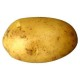# MJD

http://blog.plover.com/

I am an amateur mathematician and a professional computer programmer.

I have a blog that often carries articles about mathematics.

I wrote the book Higher-Order Perl.

Top Questions

## Proof that ${\left(\pi^\pi\right)}^{\pi^\pi}$ (and now $\pi^{\left(\pi^{\pi^\pi}\right)}$) is a noninteger.

asked May 13 '13 at 19:47

## Why does mathematical convention deal so ineptly with multisets?

asked May 31 '12 at 21:20

## Is the notorious $n^2 + n + 41$ prime generator the last of its type?

asked Jan 28 '13 at 23:08

## How can I show that $\sqrt{1+\sqrt{2+\sqrt{3+\sqrt\ldots}}}$ exists?

asked Jul 6 '13 at 3:19